Author: Oscar Cronquist Article last updated on January 25, 2019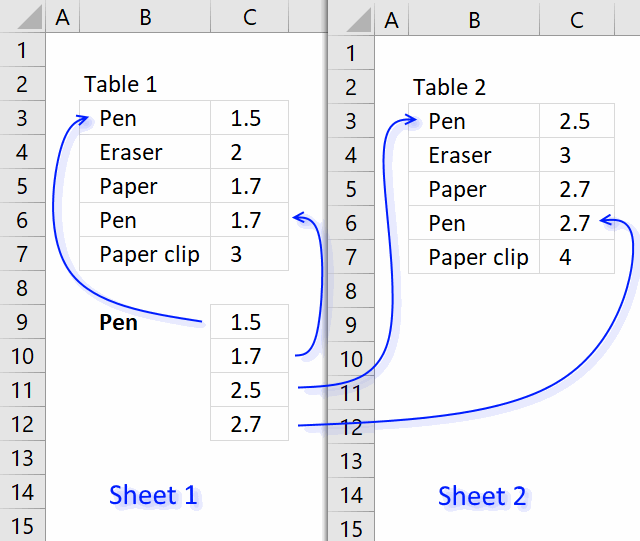This article demonstrates an array formula that searches two tables on two different sheets and returns multiple results. Sheet1 contains table1 and sheet2 contains table 2.

The search value is Pen and is in cell B9, the formula finds two matches in sheet 1 row 3 and 6. It then continues to sheet 2 and finds two matches, row 3 and 6. The adjacent values from each match is returned to cell range C9 in sheet 1.

Array Formula in cell C9:

=IFERROR(INDEX(tbl_1, SMALL(IF((\$B\$9=\$B\$3:\$B\$7), ROW(\$B\$3:\$B\$7)-MIN(ROW(\$B\$3:\$B\$7))+1, ""), ROW(A1)), 2), INDEX(tbl_2, SMALL(IF((\$B\$9=Sheet2!\$B\$3:\$B\$7), ROW(Sheet2!\$B\$3:\$B\$7)-MIN(ROW(Sheet2!\$B\$3:\$B\$7))+1, ""), ROW(A1)-SUM(--(\$B\$9=\$B\$3:\$B\$7))), 2))

### How to create an array formula

1. Copy (Ctrl + c) and paste (Ctrl + v) array formula into formula bar.
2. Press and hold Ctrl + Shift.
3. Press Enter once.
4. Release all keys.

### How to copy an array formula

1. Select cell C9
2. Copy (Ctrl + c)
3. Select cell range C9:C13
4. Paste (Ctrl + v)

### Named Range

tbl_1 - Sheet1!B3:C7
tbl_2 - Sheet2!B3:C7

How to create named range

1. Select cell range Sheet1!B3:C7
2. Type tbl_1 in name box

### Explaining array formula

#### Step 1 - What values are equal to criterion?

The equal sign lets you create a logical expression that compares cell value in B9 with values in cell range B3:B7, it creates an array containing boolean values. TRUE or FALSE.

\$B\$9=\$B\$3:\$B\$7

becomes

"Pen"={"Pen"; "Eraser"; "Paper"; "Pen"; "Paper clip"}

and returns

{TRUE; FALSE; FALSE; TRUE; FALSE}

#### Step 2 - Convert array to row numbers

The IF function has three arguments, the first one must be a logical expression. If the expression evaluates to TRUE then one thing happens (argument 2) and if FALSE another thing happens (argument 3).

If the logical expression returns TRUE the IF function replaces those values with the corresponding row numbers, if FALSE it returns "" (blank).

IF((\$B\$9=\$B\$3:\$B\$7), ROW(\$B\$3:\$B\$7)-MIN(ROW(\$B\$3:\$B\$7))+1, "")

becomes

IF({TRUE; FALSE; FALSE; TRUE; FALSE}, ROW(\$B\$3:\$B\$7)-MIN(ROW(\$B\$3:\$B\$7))+1, "")

becomes

IF({TRUE; FALSE; FALSE; TRUE; FALSE}, {3; 4; 5; 6; 7}-MIN({3; 4; 5; 6; 7})+1, "")

becomes

IF({TRUE; FALSE; FALSE; TRUE; FALSE}, {3; 4; 5; 6; 7}-3+1, "")

becomes

IF({TRUE; FALSE; FALSE; TRUE; FALSE}, {1; 2; 3; 4; 5}, "")

and returns {1; ""; ""; 4; ""}

#### Step 3 - Return the k-th smallest row number

To be able to return a new value in a cell each I use the SMALL function to filter column numbers from smallest to largest. The SMALL function ignores text and blank values in the array which is very handy in this case.

SMALL(IF((\$B\$9=\$B\$3:\$B\$7), ROW(\$B\$3:\$B\$7)-MIN(ROW(\$B\$3:\$B\$7))+1, ""), ROW(A1))

becomes

SMALL({1; ""; ""; 4; ""}, ROW(A1))

becomes

SMALL({1; ""; ""; 4; ""}, 1)

and returns 1.

#### Step 4 - Return a value or reference of the cell at the intersection of a particular row and column

The INDEX function returns a value based on a cell reference and a row number and a column number if needed.

INDEX(tbl_1, SMALL(IF((\$B\$9=\$B\$3:\$B\$7), ROW(\$B\$3:\$B\$7)-MIN(ROW(\$B\$3:\$B\$7))+1, ""), ROW(A1)), 2)

becomes

INDEX(tbl_1,1, 2)becomes

INDEX({"Pen", 1,5; "Eraser", 2; "Paper", 1,7; "Pen", 1,7; "Paper clip", 3},1, 2)

and returns \$1,5

#### Step 5 - Return another value if expression is an error

The IFERROR function returns value_if_error if expression is an error and the value of the expression itself otherwise

IFERROR(value, value_if_error)

Note, the IFERROR function catches all kinds of errors in your formula. Use this function with caution.

IFERROR function traps errors and starts looking for values in tbl_2

### Get the Excel fileVlookup-across-multiple-sheets.xlsx

### Lookup across multiple sheets Add-In

Lookup across multiple sheets is an add-in for for Excel 2007/2010/2013 (not Mac!) that lets you lookup a value or multiple values and return multiple values or rows from multiple sheets.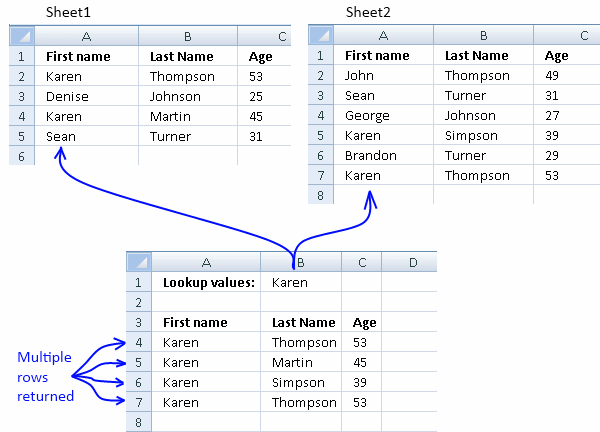### Features

• Easy-to-use custom function
• Lookups across multiple sheets
• You can use multiple search values at the same time
• Use wildcards to refine your searches even further
• Up to 127 multiple ranges or sheets
• Returns all values or rows
• Returns unique distinct values or rows
• Returns duplicate values or rows

### What you get

• Lookup across multiple sheets Add-in for Excel 2007/2010/2013 *.xlam file.
• Lookup across multiple sheets Add-in for Excel 2003 *.xla file.
• Instructions on how to install.
• Instructions on how to use custom function.
• Excel *.xlsx example file.
• Excel 2003 *.xls example file.
• 2 licenses, home and office computer.
• You can buy VBA source file for \$10 more.

### Examples

Example 1 - Lookup one value in two sheets and return multiple rows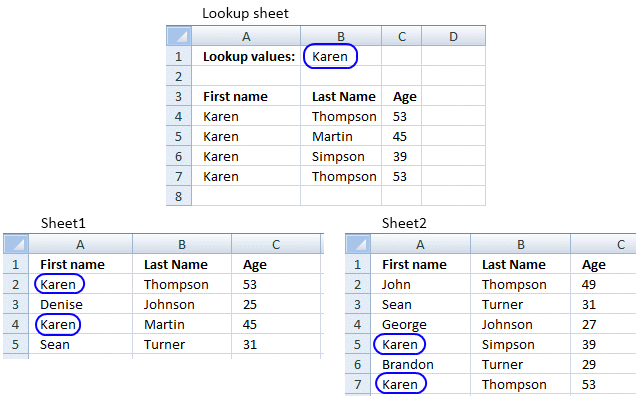Example 2 - Lookup one value in two sheets and return multiple unique distinct rows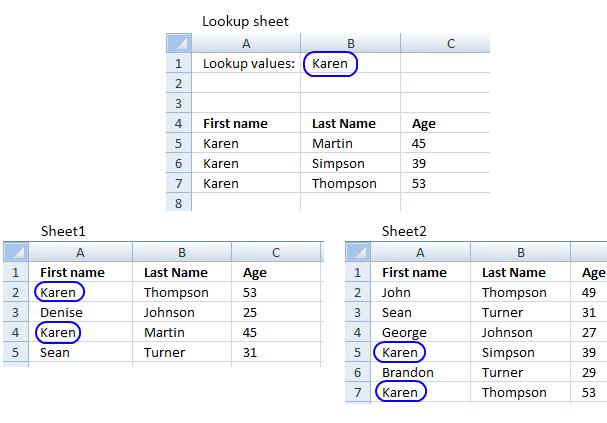Example 3 - Lookup one value in two sheets and return multiple duplicate rows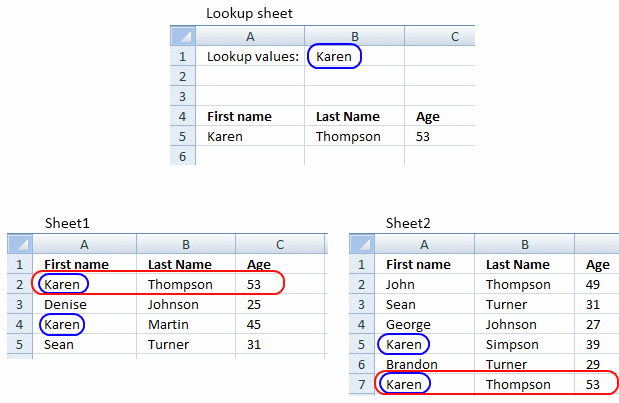Example 4 - Lookup two values in two sheets and return all matching rows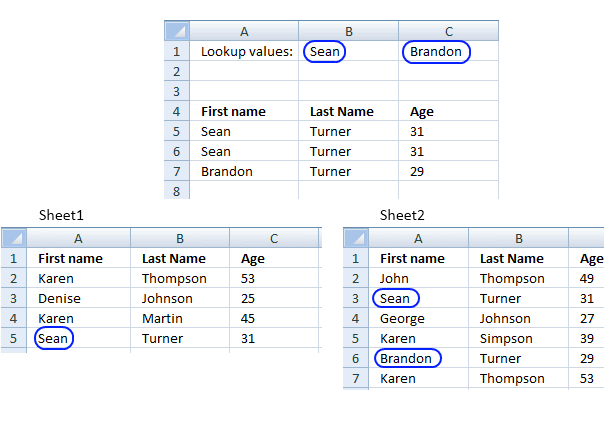Example 5 - Lookup two values in two sheets and return unique distinct rows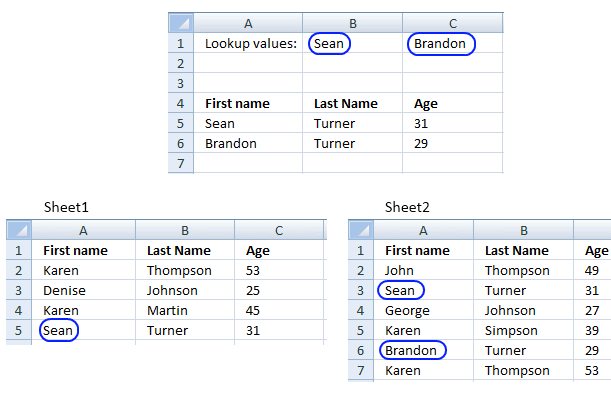Example 6 - Lookup two values in two sheets and return duplicate rowsExample 7 - Lookup two values using wildcards in two sheets and return all matching rows

Example 8 - Lookup two values using wildcards in two sheets and return multiple unique distinct rowsExample 9 - Lookup two values in two sheets using wildcards and return multiple duplicate rows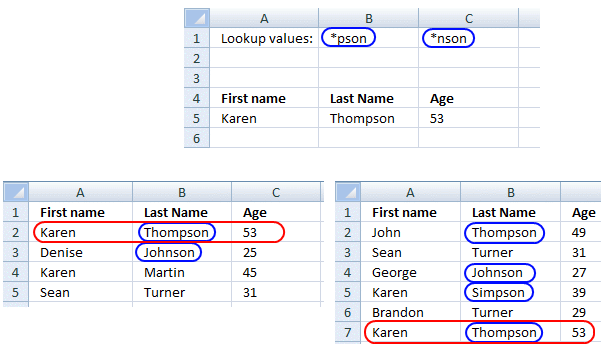Example 10 - Wildcard examples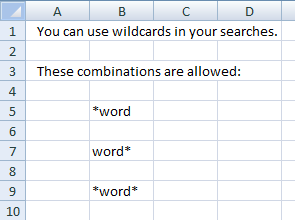How to use the custom function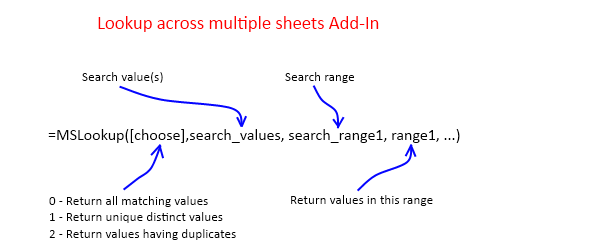### Questions:

In all your examples above you search for a value in the first column. Can the add-in search other columns?

Yes, of course! You choose which column to search, press with left mouse button on and read How to use the custom function above.

What are unique distinct values?

All values but duplicate values are removed.

Can I search case sensitive?

No, it is case insensitive.

How do I enter this user defined function?

It is an array formula.

1. Type user defined function in formula bar.
2. Press and hold Ctrl + Shift.
3. Press Enter once.
4. Release all keys.

Is there a money back guarantee?

Sure, you have un-conditional money back guarantee for 14 days.

Can I view the vba source code?

No, it is locked for viewing but you can buy VBA source file for \$40 more.

I have more questions?

Use this contact form to let me know.

Testimonials
The add-in works perfectly and it definitely saved me lots of time and effort. Thank you for answering my questions.

HL